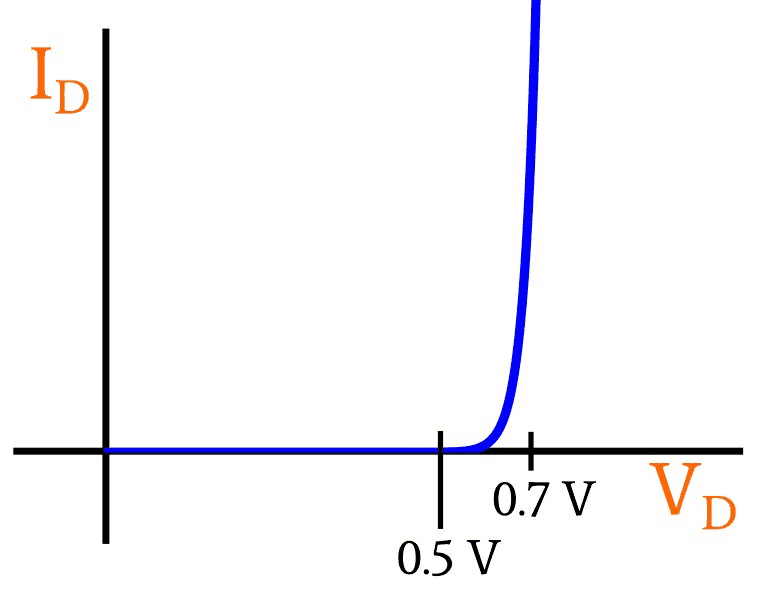# Finding the potential difference of a diode

Homework Statement:
2 Components can be connected in a circuit in series, and in parallel.

a Figure 2 shows an electrical circuit. The resistors and the (silicon) diode are connected to a power supply of negligible internal resistance.

i) Use Figure 2 to state the potential difference between points B and C when the diode is in forward bias and is conducting.

ii) Calculate the potential difference between points A and B when the diode is conducting as in part i.

iii) Use Figure 2 to calculate the current in the power supply.
Relevant Equations:
V=IR
It=I1+I2...
Sum EMF=Sum V around closed loop
Sum I in= Sum I out
1/R=1/R1 + 1/R2
I've tried doing it but i haven't been able to find the resistance of the diode.

#### Attachments

•IMG_0542.jpg
15.8 KB · Views: 107

berkeman
Mentor
Homework Statement:: 2 Components can be connected in a circuit in series, and in parallel.

a Figure 2 shows an electrical circuit. The resistors and the (silicon) diode are connected to a power supply of negligible internal resistance.

i) Use Figure 2 to state the potential difference between points B and C when the diode is in forward bias and is conducting.

ii) Calculate the potential difference between points A and B when the diode is conducting as in part i.

iii) Use Figure 2 to calculate the current in the power supply.
Relevant Equations:: V=IR
It=I1+I2...
Sum EMF=Sum V around closed loop
Sum I in= Sum I out
1/R=1/R1 + 1/R2

I've tried doing it but i haven't been able to find the resistance of the diode.
Welcome to the PF.What assumptions are you supposed to make about the forward voltage Vf of the diode? They say it is a silicon diode, so are you supposed to use a typical voltage number for it? Or are you provided a datasheet for the diode so you can use the Vf versus If plot in the datasheet to find the actual Vf when it's conducting?

•etotheipi
Welcome to the PF.What assumptions are you supposed to make about the forward voltage Vf of the diode? They say it is a silicon diode, so are you supposed to use a typical voltage number for it? Or are you provided a datasheet for the diode so you can use the Vf versus If plot in the datasheet to find the actual Vf when it's conducting?

I haven't been given a datasheet, im just wondering if there is a way for me to find the resistance of the diode, also would it be possible for the resistance of the diode to be negative? Because im not sure if i have just messed up my calculations or if it is actually possible.

berkeman
Mentor
I haven't been given a datasheet, im just wondering if there is a way for me to find the resistance of the diode, also would it be possible for the resistance of the diode to be negative? Because im not sure if i have just messed up my calculations or if it is actually possible.
For problems like this you usually don't think in terms of the "resistance" of the diode. You think instead of its forward voltage drop while conducting. For silicon diodes, you can simplify and just say Vf = 0.7V approximately, and use that in your calculation of the rest of the circuit. For example, if you have a 6V voltage source connected to a resistor + diode in series, what voltage would be dropped across the resistor if you make the simplified Vf = 0.7V assumption? And what does that give you for the current through that leg of the circuit?

The next step better in such circuits is to use the datasheet for the diode to see how Vf varies with If, and use the appropriate value of Vf in the calculation. You can see from the figure below how Vf varies with If, and how it is close to 0.6V to 0.7V for a range of If values...•cnh1995 and etotheipi
it says in my textbook that the threshold P.d. of a silicon diode is around 0.7V so it that where it comes from and is that something that i would have to assume then? so would that mean the P.d. in the diode (between b and c) is 0.7V, would that mean that the P.d. of R1 is 5.3V and the current flowing though that branch is 0.0176A?

•berkeman
cnh1995
Homework Helper
Gold Member
it says in my textbook that the threshold P.d. of a silicon diode is around 0.7V so it that where it comes from and is that something that i would have to assume then? so would that mean the P.d. in the diode (between b and c) is 0.7V, would that mean that the P.d. of R1 is 5.3V and the current flowing though that branch is 0.0176A?
Yes.

•berkeman
Ok thank you for your help